# Feed to hay

How many tons of water do we have to dehydrate from 8 tons of feed, if the original moisture is 54 percent, to get hay with a moisture content of 15 percent? Round to two decimal places.

x =  3.67 t

### Step-by-step explanation: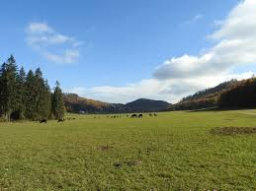Did you find an error or inaccuracy? Feel free to write us. Thank you!Tips to related online calculators
Tip: Our volume units converter will help you with the conversion of volume units.
Do you want to round the number?

## Related math problems and questions:

• Shen'sShen's chocolate bar is 54% cocoa. If the weight of the chocolate bar is 54 grams, how many grams of cocoa does it contain? Round your answer to the nearest tenth.
• CuSO4 mixtureHow many grams of solid CuSO4 we have to add to 450g of 15% CuSO4 solution to produce a 25% solution?
• 15% of15% of the revenue from sales was CZK 24,000 and it had to be written off as sales tax. What was the net profit on sales?
• Drying apples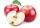By drying, apples lose 85% of their weight. How many kgs of dried apples do we get from 820 kilograms of fresh?
• Volume increaseHow many percent will increase in the pool 50 m, width 15m if the level rises from 1m to 150cm?
• Ten cashiers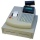Ten cashiers are open at Tesco. Customers wait an average of 15 minutes. How many other cashiers have to open to reduce the waiting time by 4 minutes?
• Wood harvestingThe harvested wood is bundled from the forest to a sawmill. The driver makes the journey four times a day, and the work takes him 8 days. How many times would he have to go daily to get the job done 2 days earlier?
• Sixty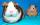Sixty percent of one-fifteen of the total is equal to thirty. What are two percent of the total?
• 14 percentIf 14 percent is 56, what is the base?
• Alcohol mixtureThree liters of 96 percent alcohol set up a certain amount of distilled water to form 54-percent alcohol. How many liters of distilled water was used?
• Apples 2Dried apples contain 15% water. Fresh apples contain 80% water. How many kg of apples we need buy in order to get 3 kg of dried apples?
• Water percent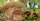A 15 g sample of the substance contains 70% water. After drying, the substance reduced the weight to 9 g. What percentage of water is now in the sample?
• Distilled waterSea water contains 5% salt. How many distilled water should be pour to 40 kg of sea water so that the salt content is 2%. How many kilograms of 2% of sea water we get?
• Pumps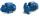Four identical pumps fill the tank in 40 hours. How many pumps would we have to use if we wanted to save 8 hours?Calculate the content of a regular 15-sides polygon inscribed in a circle with radius r = 4. Express the result to two decimal places.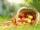How many kilograms of fresh apples id needed to 120 kg of dried apples when from 0.4 tons of fresh apples we get 75 kg of dried apples?How many grams of 5% solution do we need to add to 100g of 50% solution to get a 20% solution?